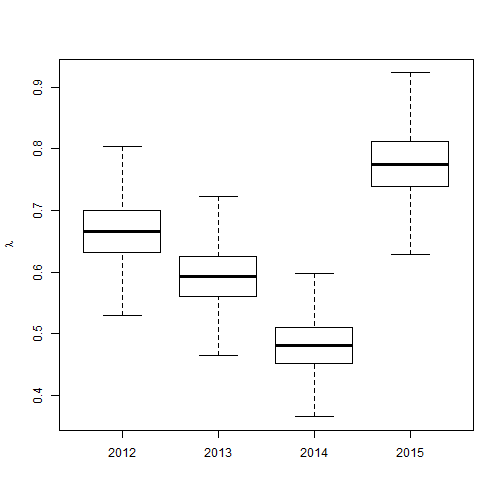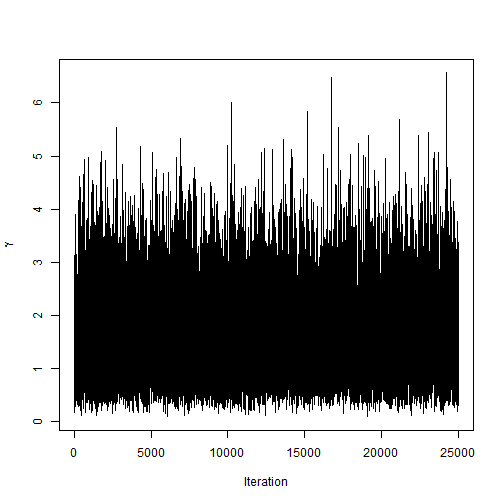# Gibbs sampling for the concussions data

### Chapter 3.2.1: Gibbs sampling

Let $$Y_i$$ be the number of concussions (aggregated over all teams and games) in season $$i$$ (1=2012,…,4=2015). We model these counts as $Y_i\sim\mbox{Poisson}(N\lambda_i) \mbox{ where } \lambda_i|\gamma\sim\mbox{Gamma}(1,\gamma),$ $$\lambda_i$$ is the concussion rate in year $$i$$ and $$N$$ is the number of games in each year. The prior for $$\gamma$$ is $$\gamma\sim\mbox{Gamma}(a,b)$$. The objective is to deterimine if the concussion rate has changed over time by comparing the posteriors of the $$\lambda_i$$.

Gibbs sampling cycles through the parameters and updates each using a draw from its full conditional distributions. The full conditional distributions are $\lambda_i|\mbox{rest}\sim\mbox{Gamma}(Y_i+1,N+\gamma)$ and $\gamma|\mbox{rest}\sim\mbox{Gamma}(a+4,b+\sum_{i=1}^4\lambda_i).$ This produces draws from the joint posterior of $$(\lambda_1,…,\lambda_4,\gamma)$$.

To evaluate whether the rate has changed between years $$i$$ and $$j$$, we approximate the posterior probabilities that $$\lambda_i>\lambda_j$$ using the proportion of the MCMC samples for which this is the case.

## Load the data

 Y <- c(171, 152, 123, 199)
n <- 4
N <- 256


## Gibbs sampling

 # Create an empty matrix for the S MCMC samples

S                 <- 25000
samples           <- matrix(NA,S,5)
colnames(samples) <- c("lam1","lam2","lam3","lam4","gamma")

# Initial values

lambda <- log(Y/N)
gamma  <- 1/mean(lambda)

# priors: lambda|gamma ~ Gamma(1,gamma), gamma ~ InvG(a,b)

a      <- 0.1
b      <- 0.1

# Gibbs sampling

for(s in 1:S){
for(i in 1:n){
lambda[i] <- rgamma(1,Y[i]+1,N+gamma)
}
gamma       <- rgamma(1,a+4,b+sum(lambda))
samples[s,] <- c(lambda,gamma)
}


## Summarize the posterior

 boxplot(samples[,1:4],outline=FALSE,ylab=expression(lambda),names=2012:2015)plot(samples[,5],type="l",xlab="Iteration",ylab=expression(gamma))# Posterior mean, median and 95% credible intervals
round(apply(samples,2,mean),2)

##  lam1  lam2  lam3  lam4 gamma
##  0.67  0.59  0.48  0.78  1.57

 round(apply(samples,2,quantile,c(0.500,0.025,0.975)),2)

##       lam1 lam2 lam3 lam4 gamma
## 50%   0.67 0.59 0.48 0.78  1.45
## 2.5%  0.57 0.50 0.40 0.67  0.44
## 97.5% 0.77 0.69 0.57 0.89  3.40


## Approximate $$\mbox{Prob}(\lambda_i>\lambda_j|Y)$$ for all pairs of $$i$$ and $$j$$

 # Is the rate higher in 2015 than 2012?
mean(samples[,4]>samples[,1])

##  0.93016

 # Is the rate higher in 2014 than 2012?
mean(samples[,3]>samples[,1])

##  0.0022

 # Is the rate higher in 2013 than 2012?
mean(samples[,2]>samples[,1])

##  0.14612

 # Is the rate higher in 2015 than 2013?
mean(samples[,4]>samples[,2])

##  0.99308

 # Is the rate higher in 2014 than 2013?
mean(samples[,3]>samples[,2])

##  0.04076

 # Is the rate higher in 2015 than 2014?
mean(samples[,4]>samples[,3])

##  0.99996


There appear to be some differences between years, but no clear increasing or decreasing trend.